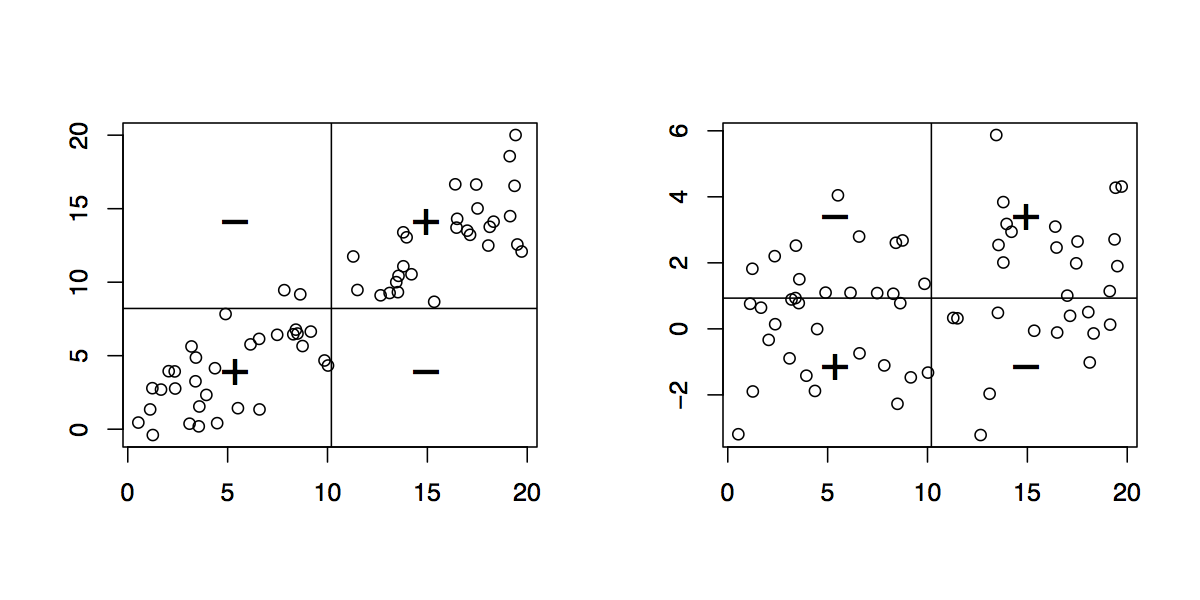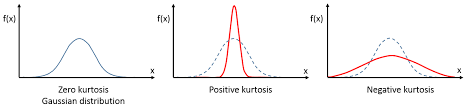# Analysis

### An interactive exploration

Teatime by Benedikt Ehinger

## The problem

• \begin{aligned} A &=& sin(2*\theta) \\B &=& sin(\theta) \end{aligned}
• \begin{aligned} C &=& 2*A &+ 0.3*B \\D &=& 2.73*A &+ 2.41*B \end{aligned}

• Undo the Stretching
• Undo the Rotation

## More formal

(and a different example)

• $$x = As$$   with   A = \left(\begin{align}\begin{array}{lr}2 & 0.3 \\ 2.73 & 2.41 \end{array}\end{align}\right)
• $\text{cov}(C,D) \overset{!}{=} I$
• $A^{-1}x = s$$\text{cov}(x,y)=\frac{1}{n}\sum_{i=1}^n(x_i-\bar x)(y_i-\bar y)$

## Excourse: How does PCA solve this problem?

• \begin{aligned} A &=& sin(2*\theta) \\B &=& sin(\theta) \end{aligned}
• \begin{aligned} C &=& 2*A &+ 0.3*B \\D &=& 2.73*A &+ 2.41*B \end{aligned}
• Undo the Stretching
• Undo the Rotation

## Two Popular Ways to solve the IC Problem

### Mutual Information

Mutual information is the amount of information that you get about the random variable X when you already know Y. It is a measure of shared information.
If X and Y are independent, mutual information is 0.Therefore by minimizing the mutual information between mixed signals, we make them independent

### Maximizing Non-Gaussianity

Central Limit Theorem: Mixtures of variables are 'more' normaly distributed. Therefore independent signals are (usually) less normal!Maximize the non-gaussianity by e.g. maximizing kurtosis, or maximizing negentropy (fastICA)

## Main Limitations

### Small Reminder:

$x = As$

x = mixed Signal, A = Mixing Matrix, s = sources

$A = WS$

W = weight Matrix, S = sphering Matrix

## Order of Sources

Sources are ordered 'randomly'. Every permutation P of s $s_{p} = Ps$ can be matched by a permuted mixingmatrix $A_{p} = AP^{-1}$ resulting in $x_{p} = A_{p}s_{p} = AP^{-1}Ps = As = x$

## Scaling of Sources

Arbitray scaled sources $$s_{k} = k*s$$: $x = As_{k}$ allows us to be matched with $A_{k} = \frac{1}{k}A$ resulting in $x = \frac{1}{k}As_{k} = \frac{k}{k}As$ this is also true for the sign ($$k = -1$$)

## Gaussian Variables

A mixture of two gaussian variables is point symmetric, it generally cannot be separated.

Maximally one gaussian variable can be separated from other non-gaussian sources using ICA

## Orthogonality

In difference to PCA, the complete mixing matrix $$A$$ is usually not orthogonal. The weight matrix W usually is, if whitening (with S) is performed.

## Assumptions

1. Each source is statistical independent from the others
2. The mixing matrix is square and of full rank, i.e. there must be the same number of signals to sources and they must be linearly independent from each other
3. There is no external noise - the model is noise free
4. The data are centered
5. There is maximally one gaussian source.